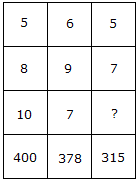# Verbal Reasoning - Character Puzzles - Discussion

### Discussion :: Character Puzzles - Character Puzzles 1 (Q.No.33)

33.

Which one will replace the question mark ?[A]. 9 [B]. 5 [C]. 7 [D]. 3

Explanation:

5 x 8 x 10 = 400

and 6 x 9 x 7 = 378

Therefore 5 x 7 x ? = 315

? = 9.

 Shoeb said: (Nov 24, 2016) I think the answer is 7. How it is 9?